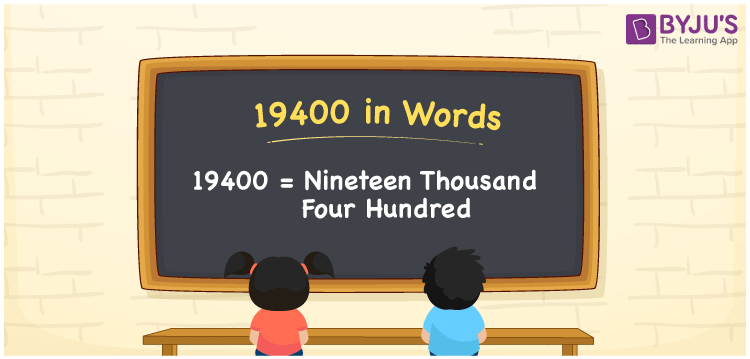# 19400 in Words

The number 19400 in words is expressed as Nineteen Thousand Four Hundred. For example, if you purchased a mobile phone worth Rs.19400, you can say, “I purchased a mobile phone worth Rupees Nineteen Thousand Four Hundred”. The number 19400 is a cardinal number since it represents a certain amount. In this article, we will learn how to write and read the number 19400 in English along with its interesting facts.

 19400 in Words Nineteen Thousand Four Hundred Nineteen Thousand Four Hundred in numerical form 19400

## 19400 in English Words

We write the numbers in words using the English alphabet as English is the most commonly used language. Hence, we can write the number 19400 in English as Nineteen Thousand Four Hundred.## How to Write 19400 in Words?

This section explains how to write a numerical name using a place value system. 19400 is a five-digit number. Hence, we should prepare a table of 5 columns. The following is the place value chart for the number 19400.

 Ten Thousand Thousand Hundreds Tens Ones 1 9 4 0 0

Hence, we can write the expanded form as:

1 x Ten Thousand + 9 x Thousand + 4 x Hundred + 0 x Ten + 0 x One

= 1 x 10000 + 9 x 1000 + 4 x 100 + 0 + 0

= 10000 + 9000 + 400

= 19400

= Nineteen Thousand Four Hundred

Therefore, 19400 in words is written as Nineteen Thousand Four Hundred

Interesting way of writing 19400 in words

1 = One

19 = Nineteen

194 = One Hundred and Ninety-Four

1940 = One Thousand Nine Hundred Forty

19400 = Nineteen Thousand Four Hundred

Thus, the word form of the number 19400 is Nineteen Thousand Four Hundred

19400 is a natural number that precedes 19401 and succeeds 19399

• 19400 in words – Nineteen Thousand Four Hundred
• Is 19400 an odd number? – No
• Is 19400 an even number? – Yes
• Is 19400 a perfect square number? – No
• Is 19400 a perfect cube number? – No
• Is 19400 a prime number? – No
• Is 19400 a composite number? – Yes

## Frequently Asked Questions on 19400 in Words

Q1

### How do you write 19400 in words?

19400 in words is written as Nineteen Thousand Four Hundred.
Q2

### Simplify 18000 + 1400, and express in words.

Simplifying 18000 + 1400, we get 19400. The number 19400 in words is Nineteen Thousand Four Hundred.
Q3

### Is 19400 an odd number?

No, 19400 is not an odd number.